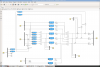Reports 1-1 of 1
VIS (General)
yoshinori.fujii - 3:50 Thursday 05 March 2020 (13335) Print this report
How about applying feed-forwarding at IP-stage?

= One suggestion for the current operation period (especially for O3 era) =

• If we can accept the low frequency drift (below ~20 mHz), we have a chance to suppress the microseismic contribution by using feed-forwarding: we can send the seismometer signal to the IP-actuators.
• Figure 1 (left): expected sensor noise coupling to the TML displacement when only the sensor-correction is used at IP-stage
• Figure 1 (right): expected sensor noise coupling to the TML displacement when both the sensor-correction and the feed-forwarding is used at IP-stage
• (black, red, blue, magenta) = (ground motion, TML (w/ control), TML (w/o control), noise coupling from IP-LVDT)
• By using both the sensor-correction and the feed-forwarding we can have further suppression compared to the case only sensor-correction is used.
• In Figure 1 (right), TML amplitude at around 150 mHz is larger than the sensor noise coupling from IP-LVDT. This is due to vertical-to-horizontal coupling (assuming the coupling ratio is 1%). In this calculation the vertical controls were not engaged. Thus we might be able to have more suppression at the frequencies comapred to Figure 1 (right).
• By using feed-forwarding, maybe we can have further suppression even if the sensor correction is imperfect.
• What I did in this simulation:
• send the output signal of the sensor correction filter to the IP-actuator after multiplying a factor as shown in Figure 2.
• An in verse of dc-gain of force transfer function is used for the factor. In the Figure 2 case, FF_IPL and FFgainIPL is set to 1.
• Figure 1: in pdf
• Figure 2: in png
Images attached to this reportNon-image files attached to this report
×

×